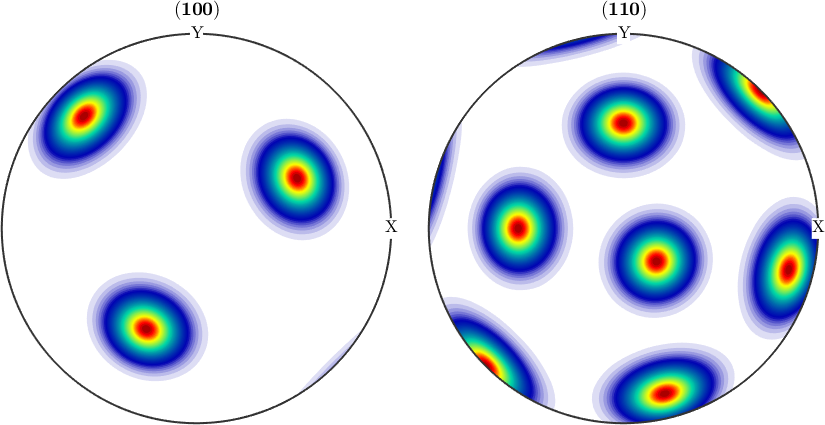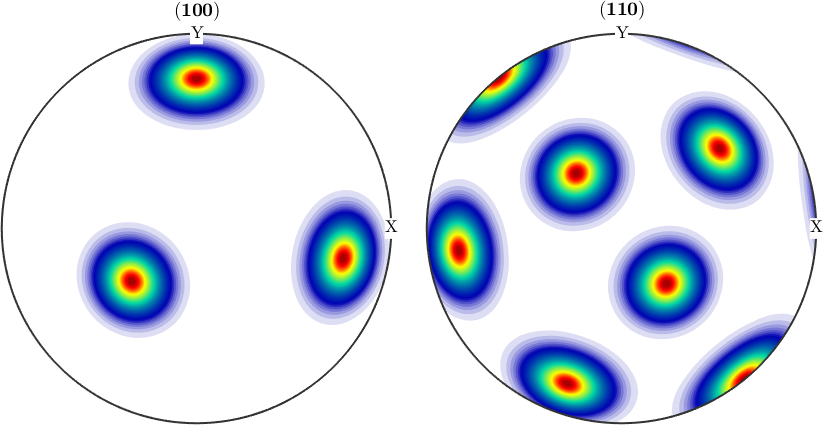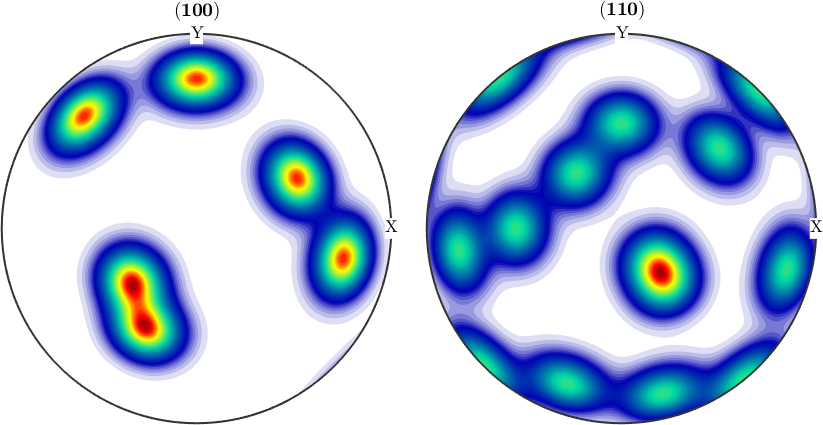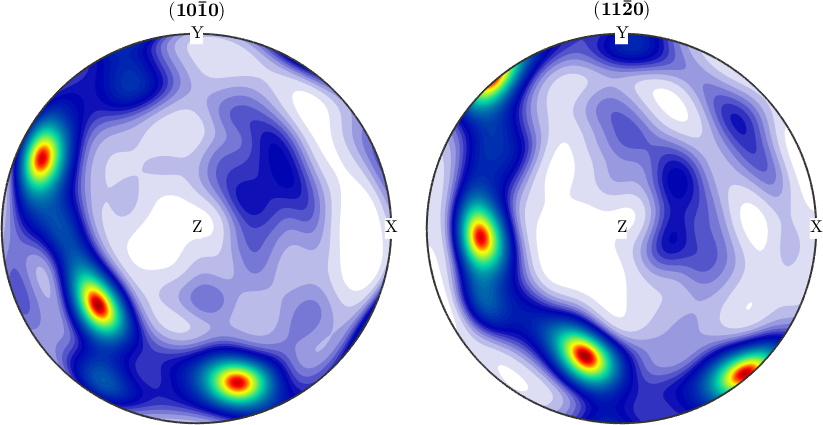Radial Basis Functions on SO(3) edit page

In MTEX we describe radial basis functions on the rotation group $$SO(3)$$ by the class SO3FunRBF.

This includes the following three types of ODFs.

## The Uniform ODF

The uniform ODF

$f(g) = 1,\quad g \in SO(3),$

is everywhere identical to one. In order to define a uniform ODF one needs only to specify its crystal and specimen symmetry and to use the command uniformODF.

## Unimodal ODFs

An unimodal ODF

$f(g; x) = \psi (\angle(g,x)),\quad g \in SO(3),$

is specified by a radial symmetrical function $$\psi$$ centered at a modal orientation, $$x\in SO(3)$$. In order to define a unimodal ODF one needsFor simplicity one can also omit the kernel function. In this case the default SO(3) de la Vallee Poussin kernel is chosen with half width of 10 degree.

## Multimodal ODFs

We define a second unimodal ODF with same kernel function and same crystal symmetry at an other orientation.By adding this unimodal ODFs we get an so called multimodal ODF, which by construction is the sum of the radial symmetrical function $$\psi$$ centered at some orientations.Its also possible to define an multimodal ODF by more than two orientations, for example